# 题目描述（中等难度）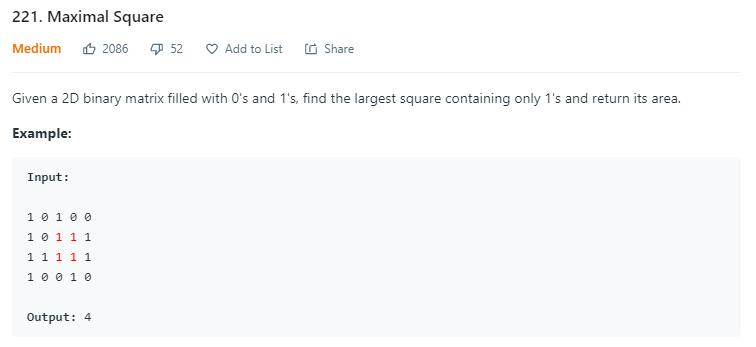# 解法一 暴力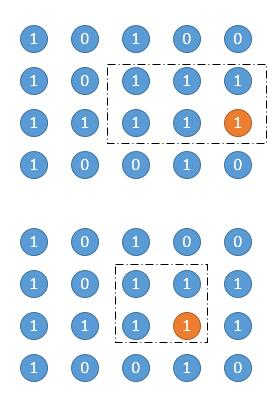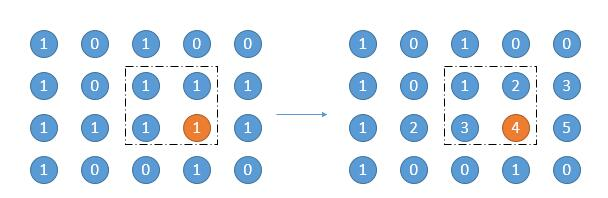1. 首先求出高度是 1 的矩形面积，也就是它自身的数，也就是上图以橙色的 4 结尾的 「1234」的那个矩形，面积就是 4。
2. 然后向上扩展一行，高度增加一，选出当前列最小的数字，作为矩阵的宽，如上图，当前列中有 24 ，那么就将 2 作为矩形的宽，求出面积，对应上图的矩形圈出的部分。
3. 然后继续向上扩展，重复步骤 2。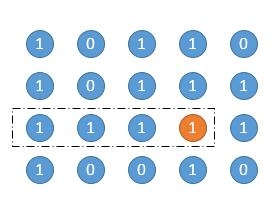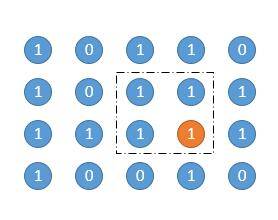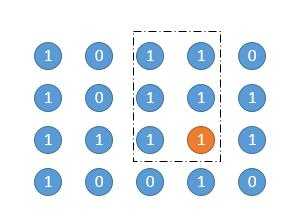public int maximalRectangle(char[][] matrix) {
if (matrix.length == 0) {
return 0;
}
//保存以当前数字结尾的连续 1 的个数
int[][] width = new int[matrix.length][matrix.length];
int maxArea = 0;
//遍历每一行
for (int row = 0; row < matrix.length; row++) {
for (int col = 0; col < matrix.length; col++) {
//更新 width
if (matrix[row][col] == '1') {
if (col == 0) {
width[row][col] = 1;
} else {
width[row][col] = width[row][col - 1] + 1;
}
} else {
width[row][col] = 0;
}
//记录所有行中最小的数
int minWidth = width[row][col];
//向上扩展行
for (int up_row = row; up_row >= 0; up_row--) {
int height = row - up_row + 1;
//找最小的数作为矩阵的宽
minWidth = Math.min(minWidth, width[up_row][col]);
//更新面积
maxArea = Math.max(maxArea, height * minWidth);
}
}
}
return maxArea;
}


public int maximalSquare(char[][] matrix) {
if (matrix.length == 0) {
return 0;
}
//保存以当前数字结尾的连续 1 的个数
int[][] width = new int[matrix.length][matrix.length];
int maxArea = 0;
/************修改的地方*****************/
int maxHeight = 0; //记录当前正方形的最大边长
/*************************************/

//遍历每一行
for (int row = 0; row < matrix.length; row++) {
for (int col = 0; col < matrix.length; col++) {
// 更新 width
if (matrix[row][col] == '1') {
if (col == 0) {
width[row][col] = 1;
} else {
width[row][col] = width[row][col - 1] + 1;
}
} else {
width[row][col] = 0;
}
// 记录所有行中最小的数
int minWidth = width[row][col];

/************修改的地方*****************/
if(minWidth <= maxHeight){
continue;
}
/*************************************/

// 向上扩展行
for (int up_row = row; up_row >= 0; up_row--) {
int height = row - up_row + 1;
// 找最小的数作为矩阵的宽
minWidth = Math.min(minWidth, width[up_row][col]);

/************修改的地方*****************/
//因为我们找正方形，当前高度大于了最小宽度，可以提前结束
if(height > minWidth){
break;
}
// 只有是正方形的时候才更新面积
if (height == minWidth) {
maxArea = Math.max(maxArea, height * minWidth);
maxHeight = Math.max(maxHeight, height);
break;
}
/*************************************/
}
}
}
return maxArea;
}


public int maximalSquare(char[][] matrix) {
if (matrix.length == 0) {
return 0;
}
// 保存以当前数字结尾的连续 1 的个数
int[][] width = new int[matrix.length][matrix.length];
// 记录最大边长
int maxSide = 0;
// 遍历每一行
for (int row = 0; row < matrix.length; row++) {
for (int col = 0; col < matrix.length; col++) {
// 更新 width
if (matrix[row][col] == '1') {
if (col == 0) {
width[row][col] = 1;
} else {
width[row][col] = width[row][col - 1] + 1;
}
} else {
width[row][col] = 0;
}
// 当前点作为正方形的右下角进行扩展
int curWidth = width[row][col];
// 向上扩展行
for (int up_row = row; up_row >= 0; up_row--) {
int height = row - up_row + 1;
if (width[up_row][col] <= maxSide || height > curWidth) {
break;
}
maxSide = Math.max(height, maxSide);
}
}
}
return maxSide * maxSide;
}


# 解法二 动态规划

dp[i][j] 表示以 matrix[i][j] 为右下角正方形的最大边长。那么递推式如下。

dp[i][j] = Min(dp[i-1][j],dp[i][j-1],dp[i-1][j-1]) + 1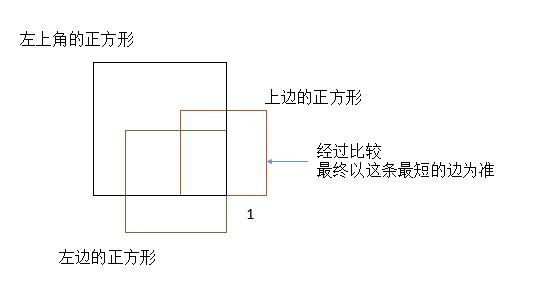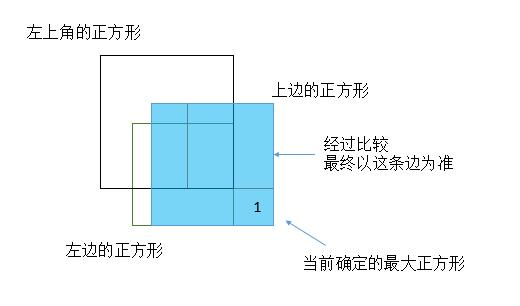public int maximalSquare(char[][] matrix) {
int rows = matrix.length;
if (rows == 0) {
return 0;
}
int cols = matrix.length;
int[][] dp = new int[rows + 1][cols + 1];
int maxSide = 0;
for (int i = 1; i <= rows; i++) {
for (int j = 1; j <= cols; j++) {
//因为多申请了一行一列，所以这里下标要减 1
if (matrix[i - 1][j - 1] == '0') {
dp[i][j] = 0;
} else {
dp[i][j] = Math.min(dp[i - 1][j], Math.min(dp[i][j - 1], dp[i - 1][j - 1])) + 1;
maxSide = Math.max(dp[i][j], maxSide);
}
}
}
return maxSide * maxSide;
}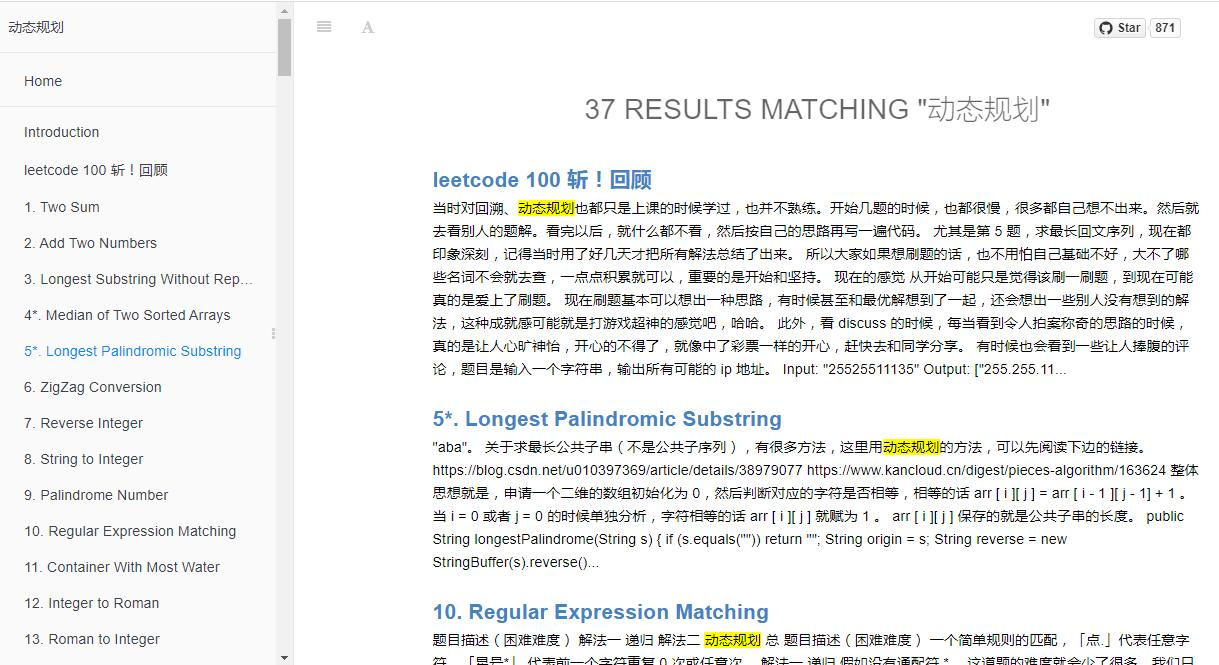public int maximalSquare(char[][] matrix) {
int rows = matrix.length;
if (rows == 0) {
return 0;
}
int cols = matrix.length;
int[] dp = new int[cols + 1];
int maxSide = 0;
int pre = 0;
for (int i = 1; i <= rows; i++) {
for (int j = 1; j <= cols; j++) {
int temp = dp[j];
if (matrix[i - 1][j - 1] == '0') {
dp[j] = 0;
} else {
dp[j] = Math.min(dp[j - 1], Math.min(dp[j], pre)) + 1;
maxSide = Math.max(dp[j], maxSide);
}
pre = temp;
}
}
return maxSide * maxSide;
}